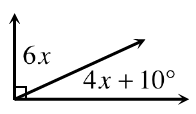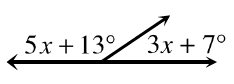### Home > GB8I > Chapter 3 Unit 4 > Lesson INT1: 3.1.2 > Problem3-21

3-21.

For each diagram below, solve for x. Explain what angle properties or relationship(s) you used for each problem.

1.• The sum of the two angles is how many degrees?

1.• The sum of the two angles is how many degrees?